Teaching support from the UK’s largest provider of in-school maths tuitionone to one lessonsschools supported

Built by teachers for teachers

In-school online one to one maths tuition developed by maths teachers and pedagogy experts

Find out more

Hundreds of FREE online maths resources!

Daily activities, ready-to-go lesson slides, SATs revision packs, video CPD and more!Explore all resources
Maths Mastery

Built by teachers for teachers

In-school online one to one maths tuition developed by maths teachers and pedagogy experts

Find out more

# White Rose Maths Year 4: What Students Learn And The Resources To Support Them

Here we look at the White Rose Maths Year 4 scheme of learning, the topics it covers across the year and provide lots of sample questions and maths resources for those following White Rose in Year 4.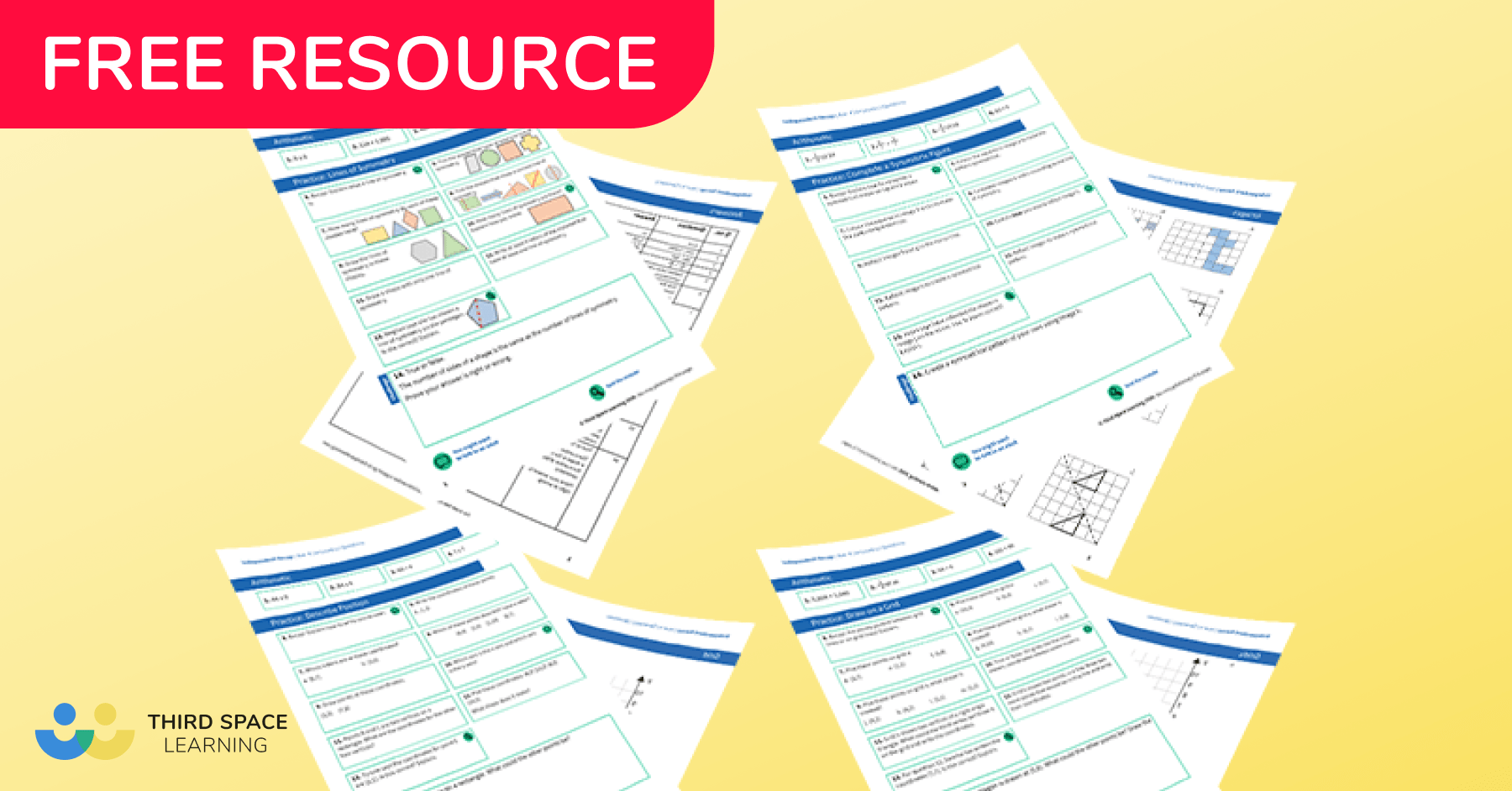Position and Coordinates Worksheets Year 4Download Free Now!

The White Rose Maths scheme of learning follows the national curriculum with a mastery approach to maths with a range of fluency, reasoning and problem solving elements. They also encourage concrete, pictorial and abstract representations to develop deep understanding of mathematical concepts.

It is thought that approximately 70% of schools in the UK follow the White Rose Maths scheme and this is reflected in the thousands of students Third Space Learning tutors every week. Our tutors are highly skilled maths and pedagogy experts, well trained in the language, representations and approach used by White Rose. They are therefore able to deliver a one to one lesson that feels familiar to students who are also used to White Rose.

Throughout this article you’ll see example lesson slides and example questions from Third Space Learning’s Year 4 maths curriculum that exemplify best practice teaching to the White Rose Maths Year 4 scheme.

We’ve also provided lots of links to Year 4 White Rose Maths resources that are suitable for use for each topic. In addition to the links provided, Third Space Learning also offers libraries for White Rose Maths Year 1, White Rose Maths Year 2, White Rose Maths Year 3, White Rose Maths Year 5 and White Rose Maths Year 6.

## About the White Rose Maths Year 4 Scheme of Learning

The primary school White Rose Maths scheme of learning has gone through several iterations and versions up to the current version 3. The scheme of learning SOL is designed as guidance and it is not necessarily intended for each small step to take one lesson. As with all teaching, it is important that teachers adapt this SOL for their class/ pupils.

### Topics covered in Year 4 White Rose Maths scheme of learning work

The White Rose Maths Year 4 SOL outlines which topics are taught, and when, throughout the autumn, spring and summer terms of Year 4.

The breakdown for Version 3 of the scheme of learning is as follows

#### Year 4 autumn term

• Place value
• Area
• Multiplication and division (A)

#### Year 4 spring term

• Multiplication and division (B)
• Length and perimeter
• Fractions
• Decimals (A)

#### Year 4 summer term

• Decimals (B)
• Money
• Time
• Shape
• Statistics
• Position and direction

​​Third Space Learning has created several collections of White Rose Maths aligned resources for all primary year groups from early years through to Year 6. These resources can provide a different perspective on a topic and so help pupils spend the time needed to fully embed a concept.

Here is a summary of the different collections available for schools following Year 4 White Rose Maths, many of them free to download from our maths hub. Links to the topic specific versions are provided within each termly breakdown below:

These ready-to-go lesson slides come in the form of editable powerpoints with related worksheets that are designed for everyday teaching. The slides cover both version 2 of the White Rose scheme of learning (in blue) and version 3 of the SOL. They also include support slides that can be used to support pupils who need a little extra  pre/ post teaching to support their understanding.

Code Crackers

Our Code Crackers resources are aligned to the version 2 SOL. These are summative resources designed as a retrieval activity for the end of the place value block.

Maths Code Crackers Year 4

Pre and Post Diagnostic Questions

These tests can be used before starting the place value block to assess gaps, and after teaching the place value block to identify progress.

Diagnostic Assessments Year 4

Worked Examples

Pupils check over completed questions to identify errors (or identify correct answers). Pupils are encouraged to explain the errors they find, not just ‘mark’ work. These worked examples help to solidify understanding by getting the pupil to discuss errors and how to avoid them.

Year 4 Worked Examples

Rapid Reasoning

These reasoning problems go alongside our Fluent in Five resource. One question each week has a model answer so teachers can understand what the question is asking and how to solve it.

Rapid Reasoning Year 4

All Kinds of Word Problems

Test your class’ problem solving skills with these place value word problems.

All Kinds of Word Problems Year 4

## White Rose Maths Year 4 autumn term

At the start of term in the autumn block 1, Year 4 focuses on place value, addition & subtraction, area and multiplication & division.

### Place Value Year 4

Place value is a principal focus of mathematics teaching in Year 4, covering a four week period at the start of the autumn term. The topic progresses on from White Rose Maths Year 3, with a number of new concepts introduced to pupils for the first time. Pupils compare numbers beyond 1000; find 1000 more and less; count backwards through zero and  round numbers to the nearest 10, 100 and 1000. At this stage, pupils also practise using Roman Numerals.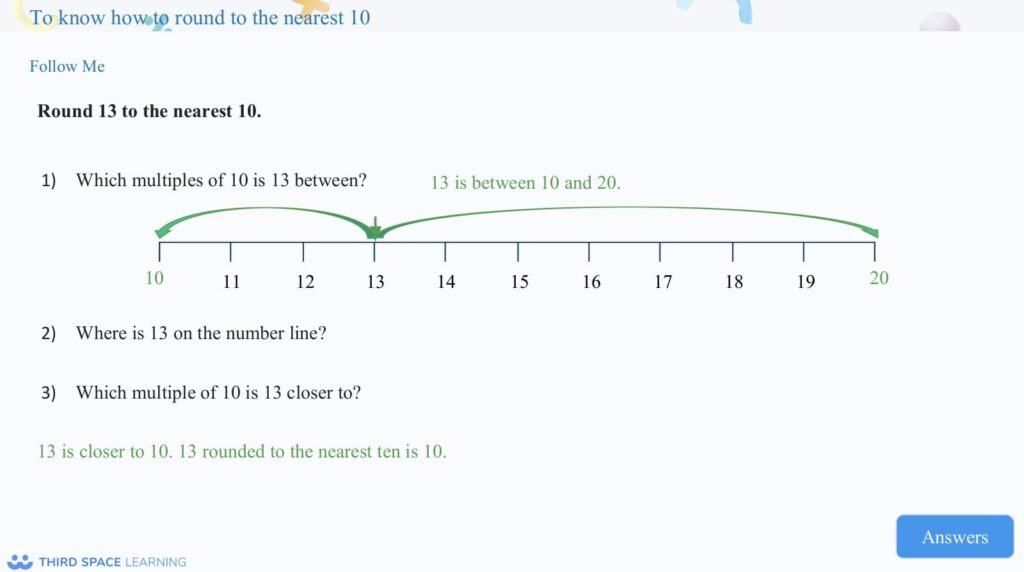An example of building upon knowledge of multiples of 10 to understand rounding.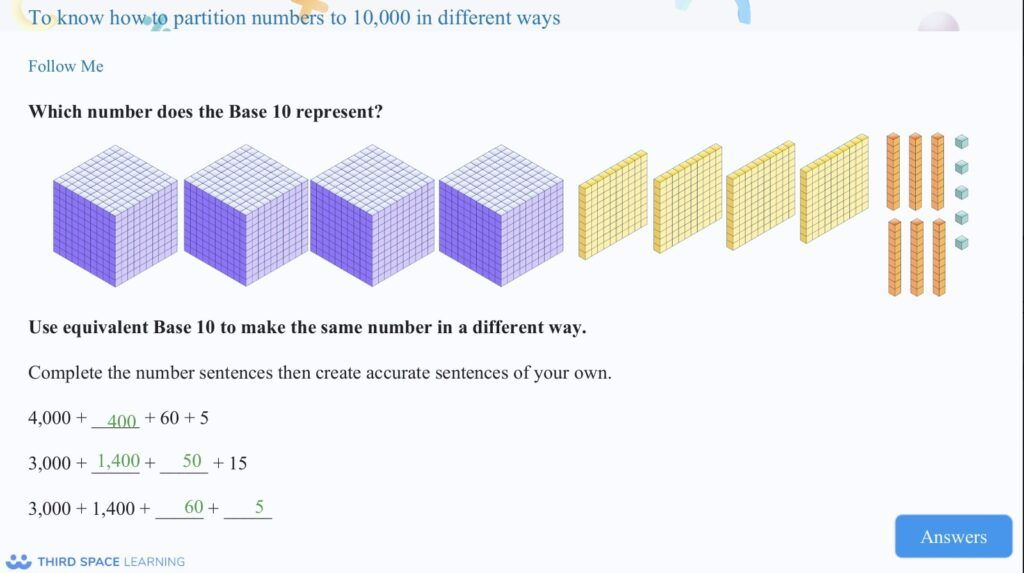This Third Space Learning slide shows using non-standard partitioning to partition a number as well as representing a number using concrete resources.

#### Example year 4 place value questions

1. What is the value of the underlined digit? 4769

2. Can you partition the number 7,358?

Answer: 7,000 + 300 + 50 + 8

3. What  number is represented by the place value counters:

### Addition and Subtraction Year 4

In year 4 during autumn block 2, children progress from adding and subtracting numbers with up to 3 digits, using a formal method, to numbers with up to 4 digits. Children continue to work with concrete resources, starting with no exchanging, then progressing to one exchange, followed by more than one exchange. They build on the problem solving skills developed in Year 3, moving  from solving one-step problems, to more complex two-step problems.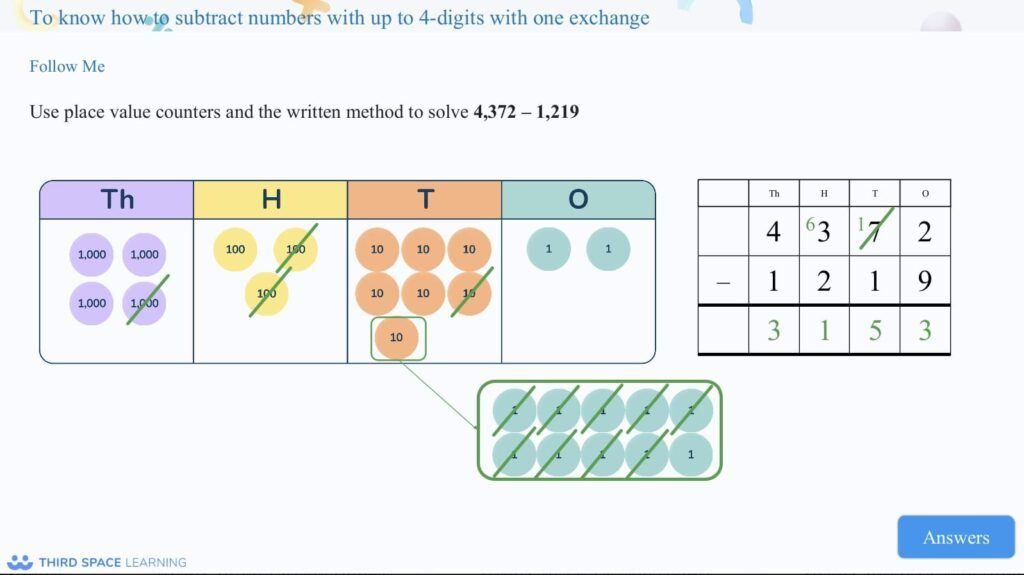This slide shows developing an understanding of exchanging using concrete resources as well as a formal written method.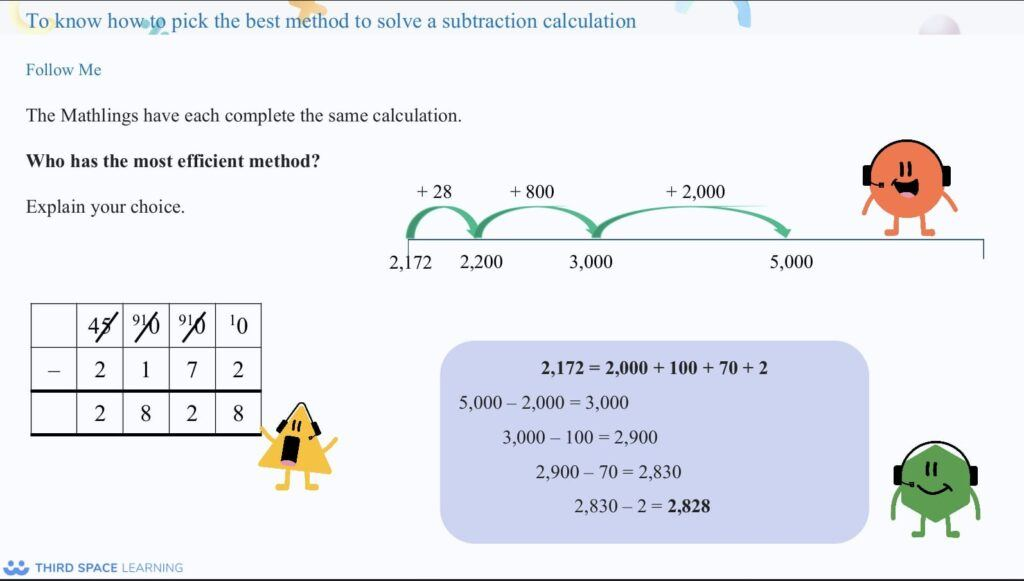It is important that pupils understand they can use different methods to solve a calculation and the written method is not always the most efficient method.

#### Example year 4 addition and subtraction questions

1. 6395 + 3867 =

2. 9342 – 4867 =

3. A pilot flew 3756km to his first destination. He then flew a further 2576km to reach his second destination. How far did he fly altogether?

### Area Year 4

In version 3 of the White Rose scheme of learning, the area topic has been taken from the spring term and has replaced length and perimeter in autumn block 3. This is a new concept in year 4, having been exposed to only perimeter in year 3. At this stage, they find the area of rectilinear shapes by counting squares.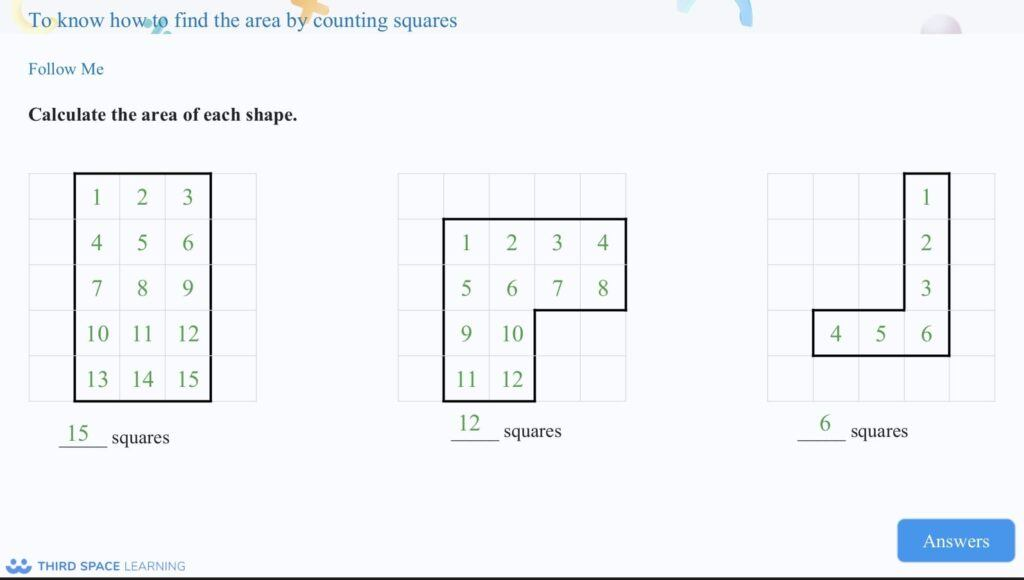Pupils learn to understand that the area is the size of the surface of a 2-D shape. In Year 4, they explore this via counting squares.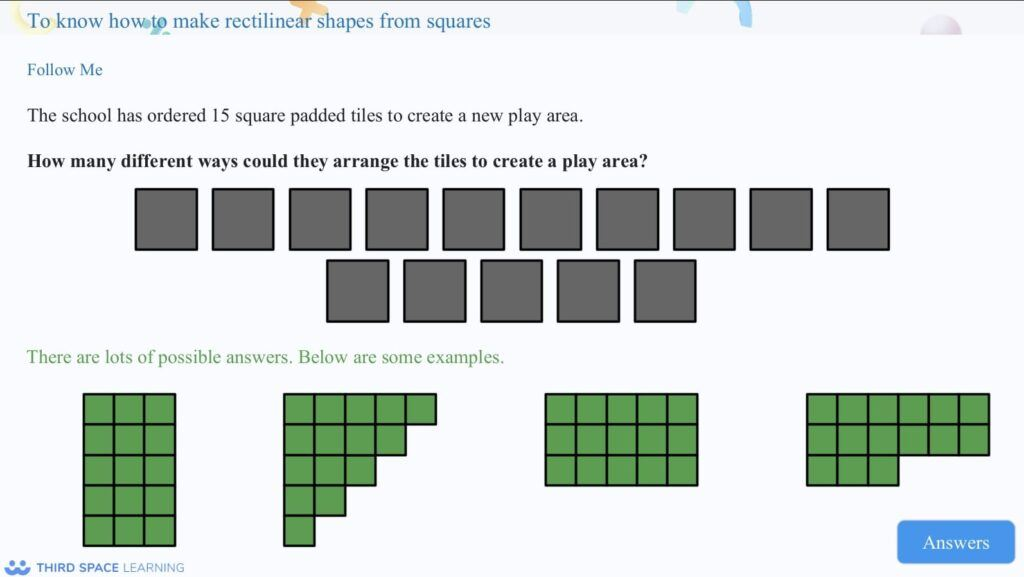Children also explore the concept that different shapes can have the same area.

#### Example year 4 area questions

1. Calculate the area of this shape (each square is 1cm)

2. Draw your own shape with an area of 11 squares

### Multiplication and Division Year 4

Multiplication and division has been split into two blocks, with block A in autumn block 4, at the end of the autumn term and block B in the spring.

### Multiplication and Division A Year 4

The first block of multiplication and division focuses on times tables knowledge; looking at multiplying and dividing. Initial focus is on the 3, 6 and 9 times tables, followed by the 7, 11 and 12 times tables. Pupils also explore multiplying and dividing by 1 and 0 and multiplying three numbers together.

#### Example year 4 multiplication and division A questions

1 . 7 × 9

2. Sam bought 7 boxes of eggs. How many eggs did he have in total?

Answer: 7 × 6 = 42

## White Rose Maths Year 4 spring term

### Multiplication and division B Year 4

This is the second block, following on from the initial multiplication and division block in the Autumn term. In this block, pupils utilise the skills developed in Block A,. Initially they investigate factor pairs and multiplying & dividing by 10 and 100, they then move on to using formal written methods to multiply 2 and 3-digit numbers by 1 digit and explore ways of partitioning 2 and 3 digits as a progression towards formal written method for division.

#### Example year 4 multiplication and division B questions

1. Calculate 365 × 4

2. 4 children participated in a sponsored swim. Each child swam 125 lengths of the pool. How many lengths did the 4 children swim in total?

Answer: 125 × 4 = 500 lengths

### Length and perimeter Year 4

In year 4, pupils build on the knowledge they gained in year 3,  progressing from finding the perimeter of rectangles, to finding the perimeter of rectilinear shapes and regular polygons. Pupils are also introduced to calculating missing lengths and to converting between units of measurement (metres and kilometres).

#### Example year 4 length and perimeter questions

1. What is the perimeter of a rectangle with a length of 7cm and a width of 4cm?

2. A park had fencing around the play equipment. The play area was 12m long and 8m wide. How many metres of fencing was used around the garden?

### Fractions Year 4

Fractions is one of the largest blocks pupils cover in year 4, spanning 4 weeks of the spring term. At this stage pupils are introduced to mixed numbers and improper fractions, and build on their understanding of equivalent fractions and adding / subtracting fractions with the same denominator. Students also begin solving problems involving increasingly harder fractions to calculate quantities, and fractions to divide quantities.

#### Example year 4 fractions questions

1. Shade in \frac{1}{3} of this rectangle:

2. \frac{3}{12} +\frac{5}{12}=

3. Mason and Ahmed baked 24 cookies. Mason ate \frac{1}{6} of the cookies and Ahmed ate \frac{1}{8}. How much did they each eat?

### Decimals Year 4

The topic on decimals in year 4 has been split into two blocks, with block A at the end of the spring term and block B in the summer.

### Decimals A Year 4

In the first decimals block, students build on their knowledge of fractions from year 3, investigating tenths and hundredths as fractions and decimals, through the use of place value charts and number lines. Pupils are also introduced to the concept of dividing 1- and 2- digit numbers by 10 and 100.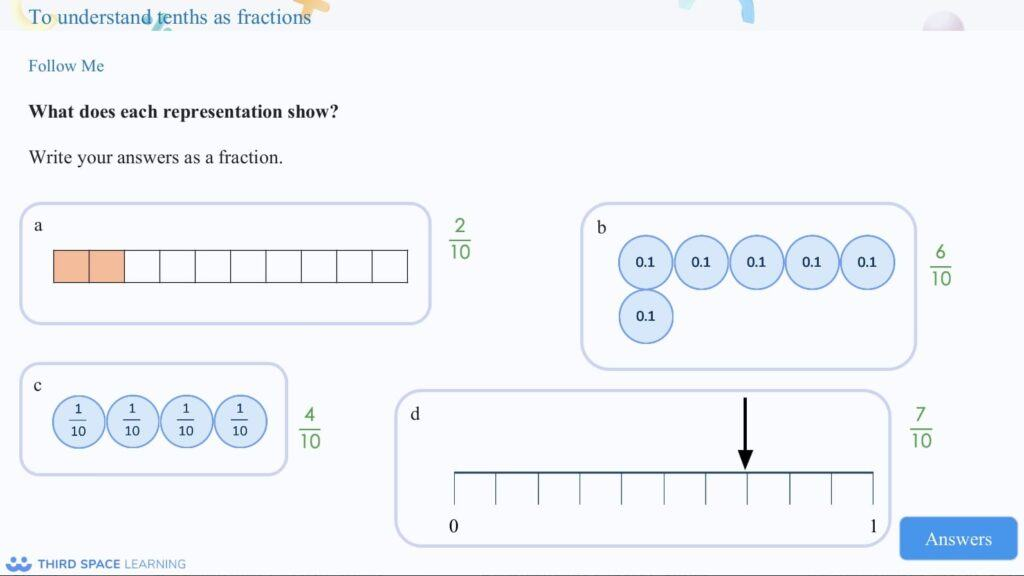Pupils will be familiar with representing integers in different ways from their place value block of work. This decimals block of work builds on this to develop an understanding of tenths as decimals.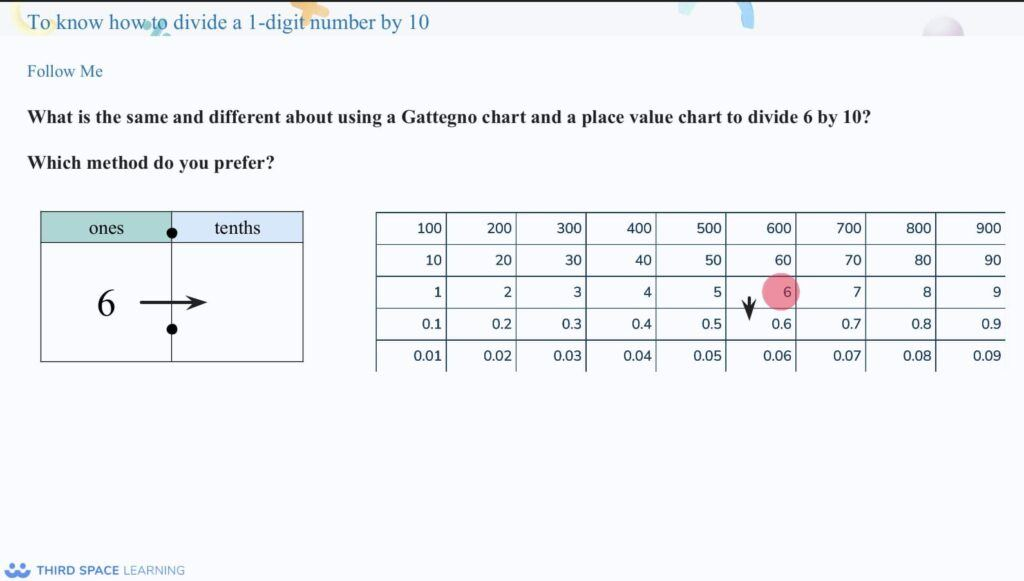Pupils use a range of methods to help them understand how to divide a 1-digit number by 10.

#### Example year 4 decimals A questions

1. Write \frac{7}{10} as a decimal

2. 42 ÷ 10 =

3. What number is being shown on the place value chart?

Show a place value chart with 5 tens, 2 ones and 6 tenths

## White Rose Maths Year 4 summer term

### Decimals B Year 4

In this second decimals unit, pupils explore making a whole with tenths and with hundredths. Students are also introduced to comparing and ordering decimals; rounding to the nearest whole number and recognising halves and quarters as decimals.

#### Example year 4 decimals B questions

1. Order the following decimal numbers from smallest to biggest

32.47     32.4        32.8    32.73

2. Round 5.58 to the nearest whole number.

3. Match the decimals and fractions below:

\frac{1}{2}0.25
\frac{3}{4} 0.1
\frac{1}{10} 0.75
\frac{1}{4}0.5

\frac{1}{2}0.5
\frac{3}{4}0.75
\frac{1}{10} 0.1
\frac{1}{4}0.25

### Money Year 4

Money is a short topic in year 4. Pupils begin by writing money using decimals, followed by converting between pounds and pence. Students also compare and estimate amounts of money and solve problems, calculating with money.

#### Example year 4 money questions

1. During a school fair, 5 of the stalls decided to work out how much money they had made. Put their totals in ascending order.

8950p     £87.23      5938p        5970p         £58.25

Answer: £58.25      5938p     5970p    £87.23      8950p

2. Chloe bought 5 cupcakes costing 45p each. If she paid with a £5 note, how much change would she get? Give your answer in pounds and pence.

### Time Year 4

By year 4, pupils should have a solid understanding of time and should be able to read, write and convert time between analogue and digital 12- and 24- hour clocks. Students need to also be able to solve problems involving converting hours to minutes, minutes to seconds, years to months and weeks to days.

#### Example year 4 time questions

1. Match the following 12 hour clock times to the correct 24 hour clock times:

3:00pm10:15
7:45am15:00
11:30pm 13:00
10:15am07:45
1:00pm23:30

3:00pm15:00
7:45am07:45
11:30pm 23:30
10:15am10:15
1:00pm13:00

2. Ahmed and Hamza went to watch a film at the cinema. The film start at 2:35pm and finished at 4:15pm. How long was the film?

Answer: 1 hour and 40 minutes

### Shape Year 4

Pupils continue to classify shapes using geometrical properties; exploring the different triangles and quadrilaterals. Knowledge of angles progresses to identifying acute and obtuse angles, and comparing and ordering angles by size. Students are introduced to symmetry in year 4; identifying lines of symmetry presented in different orientations.

#### Example year 4 shape questions

1. Sort the following angles into acute, obtuse and right angles.

• Acute: a & c
• Right angle: b
• Obtuse angle: d

2. Label the following triangles as: equilateral, isosceles or scalene:

• a) Isosceles
• b) Scalene
• c) Scalene
• d) Scalene
• e) Isosceles
• f) Equilateral

### Statistics Year 4

Statistics is one of the shortest topics of the year 4 curriculum. Pupils are taught to understand and use simple scales and interpret charts; including bar charts, pictograms and line graphs.

#### Example year 4 statistics questions

1. Sophie carried out a survey on the favourite colour of children in her year group. She represented her results on a pictogram.

a) How many children chose red as their favourite colour?

b) How many more children chose blue than green?

c) How many children did she survey altogether?

a) 10 children

b) children

c) 48 children

2. Ben measured the temperature between 7am and 2pm one day in February. He represented his results as a line graph.

a) What temperature was it at 10am?

b) What was the coldest temperature he recorded?

c) What time did the temperature start to drop again?

a) 8℃

b) 2℃

c) 1pm

### Position and Direction Year 4

Pupils read, write and use pairs of coordinates in the first quadrant. The also learn to describe movements between positions as translation up, down, left and right.

#### Example year 4 position and direction questions

1. a) On the grid above, plot the coordinates (1,2) (1,4) and (4,2)

b) If the shape is a rectangle. What would the 4th coordinate be?

a) Points plotted accurately on grid

b) The 4th coordinate would be (4,4)

2. Look at the grid below,

a) Record the current coordinates.

b) Translate ‘A’ 3 right and 2 up. Record the new coordinates.

Do you have pupils who need extra support in maths?
Every week Third Space Learning’s maths specialist tutors support thousands of pupils across hundreds of schools with weekly online 1-to-1 lessons and maths interventions designed to plug gaps and boost progress.

Since 2013 we’ve helped over 150,000 primary and secondary school pupils become more confident, able mathematicians. Learn more or request a personalised quote for your school to speak to us about your school’s needs and how we can help.

Subsidised one to one maths tutoring from the UK’s most affordable DfE-approved one to one tutoring provider.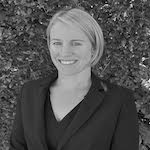##### Emma Johnson
Emma is a former Deputy Head Teacher, with 12 years' experience leading primary maths. Over the past 18 years, she has worked in primary schools in the UK and internationally, in Qatar. She now runs a tutoring company and writes resources and blogs for Third Space Learning, She is also the creator of the YouTube channel Maths4Kids with her daughter, Amber.

Related Articles

x#### Position and Coordinates Worksheets Year 4